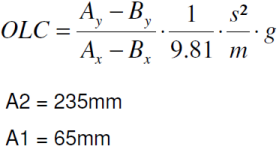# OLC

The OLC function is able to calculate both the Occupant Load Criteria (OLC) and OLC++. OLC++ combines OLC with the time of maximum deformation (Tv=0) and the sliding mean integral over a window of 25 ms (SM25), as shown in the formulas below.

## Syntax

OLC(time_vec, velocity_vec, flag, precision)

## Argument

time_vec
Time vector of the maximum deformation.
velocity_vec
Velocity vector.
flag
The return argument specifies which value(s) should be returned by the function, and has the following options:
0
OLC (the absolute value of the slope).
1
OLC++ (returns one scalar value only).
2
Returns OLC curve X (time) vector.
3
Returns OLC curve Y (velocity) vector.
4
Returns Tv0 value.
5
Returns SM25 value.
6
Returns V0 value.
7
Returns OLC, TV0, SM25, and V0 values.
precision
Define precision for the algorithm. (default = 0)
0: accuracy <= 0.1 %
1: accuracy <= 0.001 %
Optional argument.
Note: Performance could be affected at high precision.
(1)Algorithm for OLC## Note Text

High Precision

-------------

OLC = {olc(c1.x,c1.y,0,1)}

Low Precision without optional four arguments

--------------------------------------------

OLC = {olc(c1.x,c1.y,0)}

OLC++ = {olc(c1.x,c1.y,1)}

OLC curve X (time) vector = {olc(c1.x,c1.y,2)}

OLC curve Y (velocity) vector = {olc(c1.x,c1.y,3)}

Time at V=0 value = {olc(c1.x,c1.y,4)}

SM25 OLC function= {olc(c1.x,c1.y,5)}

V0 value = {olc(c1.x,c1.y,6)}

OLC, OLC++, TV0, SM25, and V0 values = {olc(c1.x,c1.y,7)}